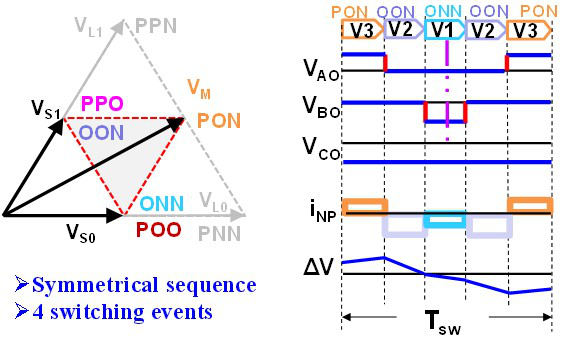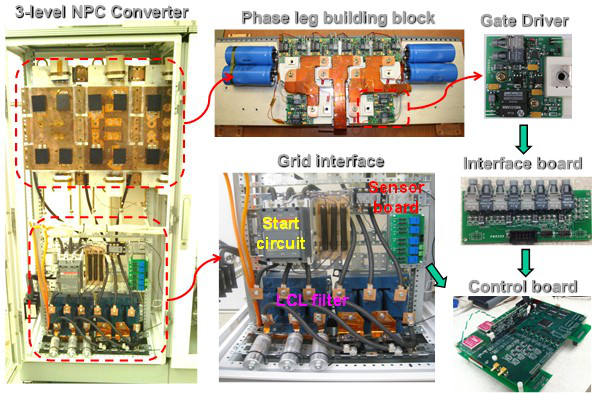LIBRARY

# Space Vector Modulation for Three-level NPC Converter with Neutral Point Voltage Balance and Switching Loss ReductionFig. 1. Pulse sequence for the proposed SVM in one switching cycle.
We have developed an improved space vector modulation (SVM) method to modulate the three-level, three-phase neutral point clamped (NPC) converter for renewable energy power conversion systems. The control objectives for the three-level NPC converter are loss reduction, neutral point (NP) balance and noise reduction of the SVM. A detailed loss model and simulation model are built to analyze quantitative loss and NP voltage ripple. An improved SVM method is proposed to simultaneously reduce the NP imbalance and the switching loss and noise.

The conventional NP balancing algorithms don't deal with the switching loss reduction because the redundant vector selection is based on a single objective. However, for high-power, high-frequency NPC power conversion systems, loss and noise reduction are important goals, in addition to the NP balance. Based on the existing control algorithms, a new SVM scheme is proposed to achieve multiple objectives at the same time.

The small vectors are selected so that both the NP charge and the pulse sequence are considered. As a result, minimized NP ripple and switching events are guaranteed within one switching cycle. The NP voltage is balanced, and the switching loss and noise are reduced. Moreover, the switching loss between switching cycles is also reduced by properly determining the pulse sequence order. This measure further reduces the total loss and, most importantly, makes symmetrical the phase leg loss distribution. The proposed SVM scheme maintains the same total loss and NP balance results under different power factors, and is verified by both simulation and experiment using the three-level NPC converter. The control result for NP balance and loss reduction are verified using a simulation model and an experimental prototype on 200 kVA three-level NPC converter hardware.Fig. 2. System structure of the three-level, three-phase NPC power converter.